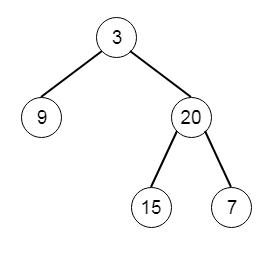# Binary Tree Zigzag Level Order Traversal in Python

Suppose we have a binary tree; we have to find the Zigzag level order traversal. So for the first row, scan from left to right, then right to left from the second row, then again left to right and so on. So if the tree is like −The traversal sequence will be [,[20,9],[15,7]]

To solve this, we will follow these steps −

• if the tree is empty, return empty list

• queue := make a queue and insert root into the queue, create two empty lists res and res2, and set flag as True

• while queue is not empty

• make a list of nodes which are present in queue, then insert into res

• make a list of node values which are present in queue, then insert into res2

• if flag is set, then

• i := length of the last sub-list in res – 1

• while i >= 0

• if ith element of the last sub-list in res has right subtree, then

• insert the right subtree into queue

• if ith element of the last sub-list in res has left subtree, then

• insert the left subtree into queue

• decrease i by 1

• otherwise

• i := length of the last sub-list in res – 1

• while i >= 0

• if ith element of the last sub-list in res has right subtree, then

• insert the right subtree into queue

• if ith element of the last sub-list in res has left subtree, then

• insert the left subtree into queue

• decrease i by 1

• return res2

Let us see the following implementation to get better understanding −

## Example

Live Demo

class TreeNode:
def __init__(self, data, left = None, right = None):
self.data = data
self.left = left
self.right = right
def insert(temp,data):
que = []
que.append(temp)
while (len(que)):
temp = que
que.pop(0)
if (not temp.left):
if data is not None:
temp.left = TreeNode(data)
else:
temp.left = TreeNode(0)
break
else:
que.append(temp.left)
if (not temp.right):
if data is not None:
temp.right = TreeNode(data)
else:
temp.right = TreeNode(0)
break
else:
que.append(temp.right)
def make_tree(elements):
Tree = TreeNode(elements)
for element in elements[1:]:
insert(Tree, element)
return Tree
class Solution(object):
def zigzagLevelOrder(self, root):
if not root:
return []
queue = [root]
res = []
res2=[]
flag = True
while len(queue)!=0:
res.append([i for i in queue])
res2.append([i.data for i in queue if i.data != 0])
queue = []
if flag:
i=len(res[-1])-1
while i>=0:
if res[-1][i].right:
queue.append(res[-1][i].right)
if res[-1][i].left:
queue.append(res[-1][i].left)
i-=1
else:
i=len(res[-1])-1
while i>=0:
if res[-1][i].left:
queue.append(res[-1][i].left)
if res[-1][i].right:
queue.append(res[-1][i].right)
i-=1
flag = not flag
return res2
ob = Solution()
tree = make_tree([3,9,20,None,None,15,7])
print(ob.zigzagLevelOrder(tree))

## Input

[3,9,20,null,null,15,7]

## Output

[, [20, 9], [15, 7]]